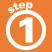Home    |    Teacher    |    Parents    |    Glossary    |    About UsIt takes two steps to solve an equation or inequality that has more than one operation:
1. Simplify using the inverse of addition or subtraction.
2. Simplify further by using the inverse of multiplication or division.Remember, when you multiply or divide an inequality by a negative number, you must reverse the inequality symbol.

Click on each equation to see how it is solved.

 Homework Help | Algebra | Equations and InequalitiesEmail this page to a friendSearch·  Solving addition and     subtraction equations·  Multiplication equations·  Division equations·  Inequalities·  Formulas·  Two-step equations     and inequalitiesFirst Glance In Depth Examples WorkoutTwo step equations and inequalities